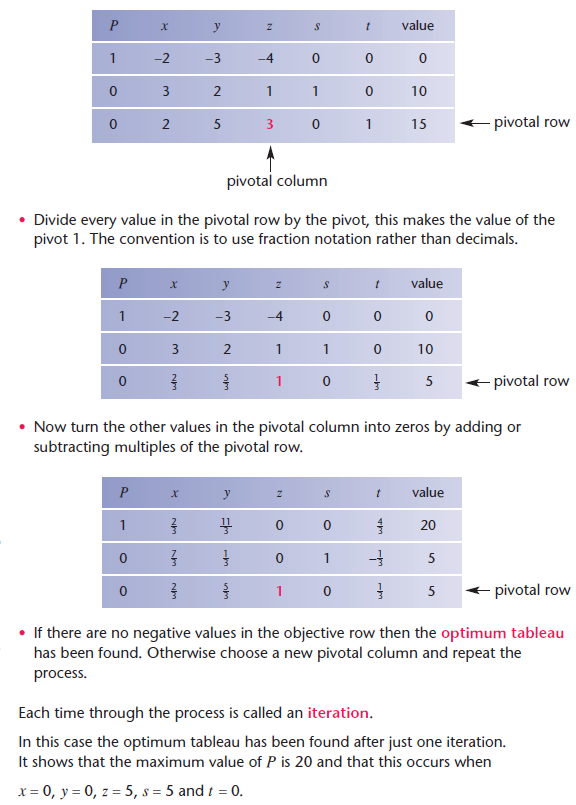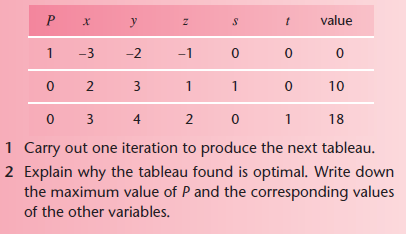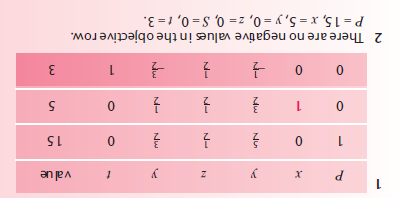## Title

Linear programming
Quick revise

After studying this section you should be able to:

• formulate a linear programming problem in terms of decision variables
• use a graphical method to represent the constraints and solve the problem
• use the Simplex algorithm to solve the problem algebraically

Formulating a linear programming problem

To formulate a linear programming problem you need to:

• Identify the variables in the problem and give each one a label.
• Express the constraints of the problem in terms of the variables. You need to include non-negativity constraints such as x ≥ 0 y ≥ 0 .
• Express the quantity to be optimised in terms of the variables. The expression produced is called the objective function.

x and y are often used for the variables. Typically, this may be to maximise a profit or minimise a loss.

Example
A small company produces two types of armchair. The cost of labour and materials for the two types is shown in the table.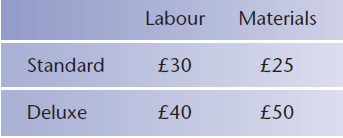The total spent on labour must not be more than £1150 and the total spent on materials must not be more than £1250. The profit on a standard chair is £70 and the profit on a deluxe chair is £100.

How many chairs of each type should be made to maximise the profit?
In this case, the variables are the number of chairs of each type that may be produced. Using x to represent the number of standard chairs and y to represent the number of deluxe chairs, the constraints may be written as:

30x + 40y ≤ 1150X ⇒ 3x + 4y ≤ 115

25x + 50y ≤ 1250X ⇒ +2y ≤ 50

and x ≥ 0,y ≥ 0.

It’s a good idea to simplify the constraints where possible.

Using P to stand for the profit, the problem is to maximise P = 70x + 100y .

The graphical method of solution

Each constraint is represented by a region on the graph. It’s a good idea to shade out the unwanted region for each one. The part that remains unshaded then defines the feasible region containing the points that satisfy all of the constraints.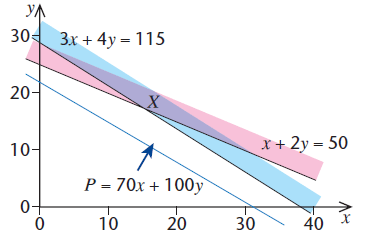The blue line represents the points where the profit takes a particular value. Moving the line in the direction of the arrow corresponds to increasing the profit. This suggests that the maximum profit occurs at the point X.

Solving 3x +4y = 115 and x +2y = 50 simultaneously gives as (15, 17.5).
The nearest points with integer coordinates in the feasible region are (15,17) and (14,18) The profit, given by P = 70x + 100y , is greater at (14,18). The maximum profit is made by producing 14 standard and 18 deluxe chairs.

X does not represent the solution in this case because both x and y must be integers.

The Simplex algorithm

When there are three or more variables, a different approach is needed. The Simplex algorithm may be used to solve the problem algebraically. The information must be expressed in the right form before the algorithm can be used.

• First write the constraints, other than the non-negativity conditions, in the form ax + by + cz ≤ d.
• Write the objective function in a form which is to be maximised.
• Add slack variables to convert the inequalities into equations.
• Write the information in a table called the initial tableau.

Example
Find the maximum value of P = 2x + 3y + 4z subject to the constraints:
3x + 2y + z ≤ 10     [i]

2x + 5y + 3z ≤ 15   [ii]

x ≥ 0,y ≥ 0, z ≥ 0.

The objective function is already in a form which is to be maximised. The constraints are in the right form.

Using slack variables s and t, the inequalities [i] and [ii] become:

3x + 2y + z + s = 10

2x + 5y + 3z + t = 15.

The objective function must be rearranged so that all of the terms are on one side of the equation to give:

P − 2x − 3y − 4z = 0.

The information may now be put into the initial tableau.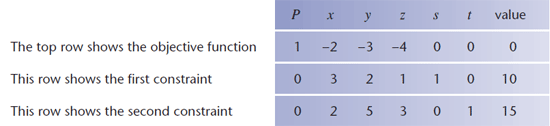The columns for P, s and t contain zeros in every row apart from one. In each case, the remaining row contains 1 and the value of the variable is given in the end column of that row. The value of every other variable is taken to be zero. So, at this stage, the tableau shows that P = 0, s = 10, t = 15 and x,y and z are all zero. This corresponds to the situation at the origin.

You need to know how to read values from the tableau.

Using the algorithm is equivalent to visiting each vertex of the feasible region until an optimum solution is found. This occurs when there are no negative values in the objective row.

When the present tableau is not optimal, a new tableau is formed as follows:

• The column containing the most negative value in the objective row becomes the pivotal column. In this case the pivotal column corresponds to the variable z.
• Now divide each entry in the value column by the corresponding entry in the pivotal column provided that the pivotal column entry is positive. For the example above this gives 10/1 = 10 and 15/3 = 5. The smallest of these results relates to the bottom row which is now taken to be the pivotal row. The entry lying in both the pivotal column and the pivotal row then becomes the pivot. In this case, the pivot is 3.